×#### Thank you for registering.

One of our academic counsellors will contact you within 1 working day.

Click to Chat

1800-1023-196

+91-120-4616500

CART 0

• 0

MY CART (5)

Use Coupon: CART20 and get 20% off on all online Study Material

ITEM
DETAILS
MRP
DISCOUNT
FINAL PRICE
Total Price: Rs.

There are no items in this cart.
Continue Shopping• Complete JEE Main/Advanced Course and Test Series
• OFFERED PRICE: Rs. 15,900
• View Details

```Chapter 7: Statistics Exercise – 7.5

Question: 1

Find the mode of the following data:

(i) 3, 5, 7, 4, 5, 3, 5, 6, 8, 9, 5, 3, 5, 3, 6, 9, 7, 4

(ii) 3, 3, 7, 4, 5, 3, 5, 6, 8, 9, 5, 3, 5, 3, 6, 9, 7, 4

(iii) 15, 8, 26, 25, 24, 15, 18, 20, 24, 15, 19, 15

Solution:

(i)

Value (x)
3
4
5
6
7
8
9

Frequency (f)
4
2
5
2
2
1
2

Mode = 5 because it occurs the maximum number of times.

(ii)

Value (x)
3
4
5
6
7
8
9

Frequency (f)
5
2
4
2
2
1
2

Mode = 3 because it occurs maximum number of times.

(iii)

Value (x)
8
15
18
19
20
24
25
26

Frequency (f)
1
4
1
1
1
2
1
1

Mode = 15 because it occurs maximum number of times.

Question: 2

The shirt size worn by a group of 200 persons, who bought the shirt from a store, are as follows:

Shirt size:
37
38
39
40
41
42
43
44

Number of persons:
15
25
39
41
36
17
15
12

Find the model shirt size worn by the group.

Solution:

Shirt size
37
38
39
40
41
42
43
44

Number of persons
15
25
39
41
36
17
15
12

Model shirt size = 40 because it occurs maximum number of times.

Question: 3

Find the mode of the following distribution. (i)

Class interval:
0 – 10
10 – 20
20 – 30
30 – 40
40 – 50
50 – 60
60 – 70
70 – 80

Frequency:
5
8
7
12
28
20
10
10

(ii)

Class interval:
10 – 15
15 – 20
20 – 25
25 – 30
30 – 35
35 – 40

Frequency:
30
45
75
35
25
15

(iii)

Class interval:
25 – 30
30 – 35
35 – 40
40 – 45
45 – 50
50 – 55

Frequency:
25
34
50
42
29
15

Solution:

(i)

Class interval
0-10
10 – 20
20 – 30
30 – 40
40 – 50
50 – 60
60 – 70
70 – 80

Frequency
5
8
7
12
28
20
10
10

Here the maximum frequency is 28 then the corresponding class 40 – 50 is the modal class

l = 40, h = 50 40 = 10, f = 28, f1 = 12, f2 = 20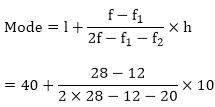= 40 + 160/ 24

= 40 + 6.67

= 46.67

(ii)

Class interval
10 – 15
15 – 20
20 – 25
25 – 30
30 – 35
35 – 40

Frequency
30
45
75
35
25
15

Here the maximum frequency is 75, then the corresponding class 20 - 25 is the modal class

l = 20, h = 25 - 20 = 5, f = 75, f1 = 45, f2 = 35= 20 + 150/70

= 20 + 2.14

= 22.14

(iii)

Class interval
25 – 30
30 – 35
35 – 40
40 – 45
45 – 50
50 – 55

Frequency
25
34
50
42
38
14

Here the maximum frequency is 50 then the corresponding class 35 - 40 is the modal class

l = 35, h = 40 - 35 = 5, f = 50, f1 = 34, f2 = 42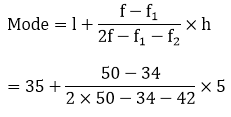= 35 + 80/24

= 35 + 3.33

= 38.33

Question: 4

Compare the modal ages of two groups of students appearing for an entrance test:

Age in years
16 – 18
18 – 20
20 – 22
22 – 24
24 – 26

Group A
50
78
46
28
23

Group B
54
89
40
25
17

Solution:

Age in years
16 – 18
18 – 20
20 – 22
22 – 24
24 – 26

Group A
50
78
46
28
23

Group B
54
89
40
25
17

For Group A Here the maximum frequency is 78, then the corresponding class 18 – 20 is model class l = 18, h = 20 - 18 = 2, f = 78, f1 = 50, f2 = 46= 18 + 56/60

= 18 + 0.93

= 18.93 years

For group B Here the maximum frequency is 89, then the corresponding class 18 - 20 is the modal class l = 18, h = 20 - 18 = 2, f = 89, f1 = 54, f2 = 40 Mode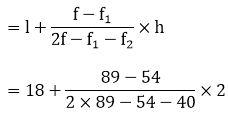= 18 + 70/84

= 18 + 0.83

= 18.83 years

Hence the modal age for the Group A is higher than that for Group B

Question: 5

The marks in science of 80 students of class X are given below. Find the mode of the marks obtained by the students in science.

Marks
0 – 10
10 – 20
20 – 30
30 – 40
40 – 50
50 – 60
60 – 70
70 – 80
80 – 90
90 – 100

Frequency
3
5
16
12
13
20
5
4
1
1

Solution:

Marks
0 – 10
10 – 20
20 – 30
30 – 40
40 – 50
50 – 60
60 – 70
70 – 80
80 – 90
90 – 100

Frequency
3
5
16
12
13
20
5
4
1
1

Here the maximum frequency is 20, then the corresponding class 50 – 60 is the modal class l = 50, h = 60 – 50 = 10, f = 20, f1 = 13, f2 = 5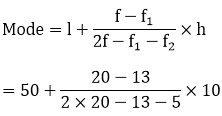= 50 + 70/22

= 50 + 3.18

= 53.18

Question: 6

The following is the distribution of height of students of a certain class in a city:

Height (in cm):
160 – 162
163 – 165
166 – 168
169 – 171
172 – 174

No of students:
15
118
142
127
18

Find the average height of maximum number of students.

Solution:

Heights(exclusive)
160 – 162
163 – 165
166 – 168
169 – 171
172 – 174

Heights (inclusive)
159.5 – 162.5
162.5 – 165.5
165.5 – 168.5
168.5 – 171.5
171.5 – 174.5

No of students
15
118
142
127
18

Here the maximum frequency is 142, then the corresponding class 165.5 – 168.5 is the modal class l = 165.5, h = 168.5 - 165.5 = 3, f = 142, f1 = 118, f2 = 127= 165.5 + 72/39

= 165.5 + 1.85

= 167.35 cm

Question: 7

The following table shows the ages of the patients admitted in a hospital during a year:

Ages (in years):
5 – 15
15 – 25
25 – 35
35 – 45
45 – 55
55 – 65

No of students:
6
11
21
23
14
5

Find the mode and the mean of the data given above. Compare and interpret the two measures of central tendency.

Solution:

We may compute class marks (xi) as per the relation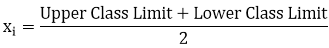Now taking 30 as assumed mean (a) we may calculate di and fidi as follows:

Age (in years)
Number of patients fi
Class marks xi
di = xi – 275
fidi

5 – 15
6
10
- 20
-120

15  25
11
20
- 10
-110

25  35
21
30
0
0

35  45
23
40
10
230

45 - 55
14
50
20
280

55  65
5
60
30
150

Total
80

430

From the table we may observe that Σfi = 80, Σfi di = 430,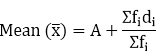= 30 + 430/80

= 30 + 5.375

= 35.375

= 35.38

Clearly, mean of this data is 35.38. It represents that on an average the age of a patients admitted to hospital was 35.38 years.

As we may observe that maximum class frequency is 23 belonging to class interval 35 – 45

So, modal class = 35 – 45 Lower limit (l) of modal class = 35 Frequency (f) of modal class = 23

Class size (h) = 10 Frequency (f1) of class preceding the modal class = 21 Frequency (f2) of class succeeding the modal class = 14 Mode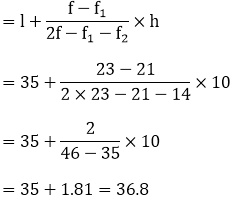Clearly mode is 36.8. It represents that maximum number of patients admitted in hospital were of 36.8 years.

Question: 8

The following data gives the information on the observed lifetimes (in hours) of 225 electrical components:

0 – 20
20 – 40
40 – 60
60 – 80
80 – 100
100 – 120

No of components:
10
35
52
61
38
29

Determine the modal lifetimes of the components.

Solution:

From the data given as above we may observe that maximum class frequency is 61 belonging to class interval 60 – 80

So, modal class limit (l) of modal class = 60 Frequency (f) of modal class = 61 Frequency (f1) of class preceding the modal class = 52

Frequency (f2) of class succeeding the modal class = 38 Class size (h) = 20 Mode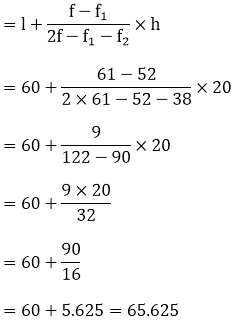So, modal lifetime of electrical components is 65.625 hours

Question: 9

The following table gives the daily income of 50 workers of a factory:

Daily income
100 – 120
120 – 140
140 – 160
160 – 180
180 – 200

Number of workers
12
14
8
6
10

Find the mean, mode and median of the above data.

Solution:

Class interval
Mid value (x)
Frequency (f)
fx
Cumulative frequency

100 – 120
110
12
1320
12

120 – 140
130
14
1820
26

140 – 160
150
8
1200
34

160 – 180
170
6
1000
40

180 – 200
190
10
1900
50

N = 50
Σfx = 7260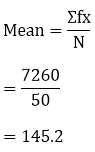We have, N = 50 Then, N/2 = 50/2 = 25

The cumulative frequency just greater than N/2 is 26, then the median class is 120 - 140 such that l = 120, h = 140 - 120 = 20, f = 14, F = 12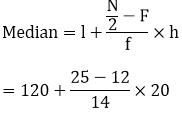= 120 + 260/14

= 120 + 18.57

= 138.57

Here the maximum frequency is 14, then the corresponding class 120 - 140 is the modal class l = 120, h = 140 - 120 = 20, f = 14, f1 = 12, f2 = 8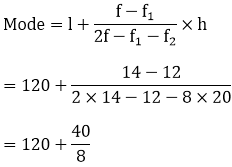= 120 + 5

= 125

Question: 10

The following distribution gives the state-wise teachers-students ratio in higher secondary schools of India. Find the mode and mean of this data. Interpret the two measures:

Number of students per teacher
Number of states/ U.T
Number of students per teacher
Number of states/ U.T

15 – 20
3
35 – 40
3

20 – 25
8
40 – 45
0

25 – 30
9
45 – 50
0

30 – 35
10
50 – 55
2

Solution:

We may observe from the given data that maximum class frequency is 10 belonging to class interval 30 – 35.

So, modal class = 30 – 35 Class size (h) = 5 Lower limit (l) of modal class = 30 Frequency (f) of modal class = 10 Frequency (f1) of class preceding modal class = 9

Frequency (f2) of class succeeding modal class = 3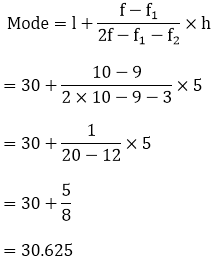Mode = 30.6 It represents that most of states/ U.T have a teacher- student ratio as 30.6 Now we may find class marks by using the relation Class mark = (upper class limit + lower class limit) /2.

Now taking 32.5 as assumed mean (a) we may calculate di, ui, and fiui as following

Number of students per teacher
Number of states/ U.T (fi)
xi
di = xi – 32.5
Ui
fiui

15 – 20
3
17.5
-15
- 3
- 9

20 – 25
8
22.5
-10
- 2
- 16

25 – 30
9
27.5
-5
- 1
- 9

30 – 35
10
32.5
0
0
0

35 – 40
3
37.5
5
1
3

40 – 45
0
42.5
10
2
0

45 – 50
0
47.5
15
3
0

50 – 55
2
52.5
20
4
8

Total
35

-23

Now,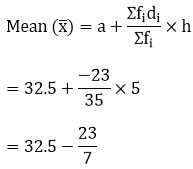So mean of data is 29.2 It represents that on an average teacher-student ratio was 29.2

Question: 11

Find the mean, median and mode of the following data:

Classes:
0 – 50
50 – 100
100 – 150
150 – 200
200 – 250
250 – 300
300 – 350

Frequency:
2
3
5
6
5
3
1

Solution:

Class interval
Mid value (x)
Frequency (f)
fx
Cumulative frequency

0 – 50
35
2
50
2

50 – 100
75
3
225
5

100 – 150
125
5
625
10

150 – 200
175
6
1050
16

200 – 250
225
5
1127
21

250 – 300
275
3
825
24

300 – 350
325
1
325
25

N = 25
Σfx = 4225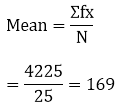We have, N = 25

Then, N/2

= 25/2

= 12.5

The cumulative frequency just greater than N/2 is 16, then the median class is 150 - 200 such that l = 150, h = 200 - 150 = 50, f = 6, F = 10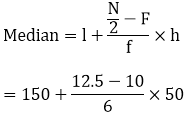= 150 + 125/6

= 150 + 20.83

= 170.83

Here the maximum frequency is 6, then the corresponding class 150 - 200 is the modal class l = 150, h = 200 - 150 = 50, f = 6, f1 = 5, f2 = 5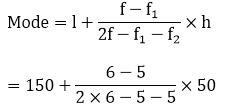= 150 + 50/2

= 150 + 25

= 175

Question: 12

A students noted the number of cars pass through a spot on a road for 100 periods each of 3 minute and summarized it in the table given below. Find the mode of the data.

Solution:

From the given data we may observe that maximum class frequency is 20 belonging to 40 – 50 class intervals.

So, modal class = 40 – 50 Lower limit (l) of modal class = 40 Frequency (f) of modal class = 20 Frequency (f1) of class preceding modal class = 12

Frequency (f2) of class succeeding modal class = 11 Class size = 10= 40 + 80/17

= 40 + 4.7

= 44.7

So mode of this data is 44.7 cars

Question: 13

The following frequency distribution gives the monthly consumption of electricity of 68 consumers of a locality. Find the median, mean and mode of the data and compare them.

Monthly consumption:
65 – 85
85 – 105
105  – 125
125 – 145
145 – 165
165 – 185
185 – 205

No of consumers:
4
5
13
20
14
8
4

Solution:

Class interval
Mid value xi
Frequency fi
fx
Cumulative frequency

65 – 85
75
4
300
4

85 – 105
95
5
475
9

105 – 125
115
13
1495
22

125 – 145
135
20
2700
42

145 – 165
155
14
2170
56

165  185
175
8
1400
64

185  205
195
4
780
68

N = 68

Σfx = 9320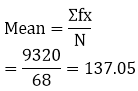We have, N = 68 N/2 = 68/2 = 34

The cumulative frequency just greater than N/2 is 42 then the median class is 125 - 145 such that l = 125, h = 145 - 125 = 20, f = 20, F = 22= 125 + 12

= 137

Here the maximum frequency is 20, then the corresponding class 125 - 145 is the modal class l = 125, h = 145 - 125 = 20, f = 20, f1 = 13, f2 = 14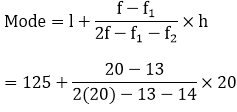= 125 + 140/13

= 135.77

Question: 14

100 surnames were randomly picked up from a local telephone directly and the frequency distribution of the number of letter English alphabets in the surnames was obtained as follows:

Number of letters:
1 – 4
4 – 7
7 – 10
10 – 13
13 – 16
16  – 19

Number surnames:
6
30
40
16
4
4

Determine the median number of letters in the surnames. Find the mean number of letters in the surnames. Also, find the modal size of the surnames.

Solution:

Class interval
Mid value (x)
Frequency (f)
fx
Cumulative frequency

1 – 4
2.5
6
15
6

4 – 7
5.5
30
165
36

7 – 10
8.5
40
340
76

10 – 13
11.5
16
184
92

13 – 16
14.5
4
58
96

16 – 19
17.5
4
70
100

N = 100
Σfx = 832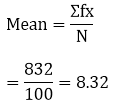We have, N = 100 N/2 = 100/2 = 50

The cumulative frequency just greater than N/2 is 76, then the median class is 7 - 10 such that l = 7, h = 10 - 7 = 3, f = 40, F = 36= 7 + 1.05

= 8.05

Here the maximum frequency is 40, then the corresponding class 7 - 10 is the modal class l = 7, h = 10 - 7 = 3, f = 40, f1 = 30, f2 = 16= 7 + 30/34

= 7 + 0.88

= 7.88

Question: 15

Find the mean, median and mode of the following data:

Class
0 - 20
20 – 40
40 – 60
60 – 80
80 – 100
100 – 120
120 – 140

Frequency
6
8
10
12
6
5
3

Solution:

Class interval
Mid value (x)
Frequency (f)
fx
Cumulative frequency

0 – 20
10
6
60
6

20 – 40
30
8
240
17

40 – 60
50
10
500
24

60 – 80
70
12
840
36

80 – 100
90
6
540
42

100 – 120
110
5
550
47

120 – 140
130
3
390
50

N = 50
Σfx = 3120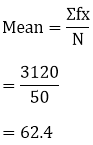We have, N = 50 Then, N/2 = 50/2 = 25

The cumulative frequency just greater than N/2 is 36, then the median class is 60 - 80 such that l = 60, h = 80 - 60 = 20, f = 12, F = 24= 60 + 20/12

= 60 + 1.67

= 61.67

Here the maximum frequency is 12, then the corresponding class 60 - 80 is the modal class l = 60, h = 80 - 60 = 20, f = 12, f1 = 10, f2 = 6Question: 16

The following data gives the distribution of total monthly household expenditure of 200 families of a village. Find the modal monthly expenditure of the families. Also, find the mean monthly expenditure:

Expenditure
Frequency
Expenditure
Frequency

1000 – 1500
24
3000 – 3500
30

1500 – 2000
40
3500 – 4000
22

2000 – 2500
33
4000 – 4500
16

2500 – 3000
28
4500 – 5000
7

Solution:

We may observe from the given data that maximum class frequency is 40 belonging to 1500 -200 intervals. So, modal class = 1500 -2000

Lower limit (l) of modal class = 1500 Frequency (f) of modal class = 40 Frequency (f1) of class preceding modal class = 24

Frequency (f2) of class succeeding modal class = 33 Class size (h) = 500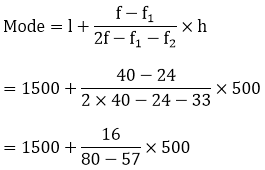= 1500 + 347.826

= 1847.826

1847.83

So modal monthly expenditure was Rs. 1847.83

Now we may find class mark as Class markClass size (h) of given data = 500 Now taking 2750 as assumed mean (a) we may calculate di ui as follows:

Expenditure (in Rs)
Number of families fi
xi
di = xi – 2750
Ui
fiui

1000 – 1500
24
1250
- 1500
- 3
- 72

1500 – 2000
40
1750
- 1000
- 2
- 80

2000 – 2500
33
2250
- 500
- 1
- 33

2500 – 3000
28
2750
0
0
0

3000 – 3500
30
3250
500
1
30

3500 – 4000
22
3750
1000
2
44

4000 – 4500
16
4250
1500
3
48

4500 – 5000
7
4750
2000
4
28

Total
200

-35

Now from table may observe that Σfi = 200, Σfidi = -35So mean monthly expenditure was Rs. 2662.5

Question: 17

The given distribution shows the number of runs scored by some top batsmen of the world in one day international cricket matches.

Runs scored
No of batsmen
Runs scored
No of batsmen

3000 – 4000
4
7000 – 8000
6

4000 – 5000
18
8000 – 9000
3

5000 – 6000
9
9000 – 10000
1

6000 – 7000
7
10000 – 11000
1

Find the mode of the data.

Solution:

From the given data we may observe that maximum class frequency is 18 belonging to class interval 4000 – 5000.

So, modal class = 4000 – 5000 Lower limit (l) of modal class = 4000

Frequency (f) of modal class = 18. Frequency (f1) of class preceding modal class = 4 Frequency (f2) of class succeeding modal class = 9 Class size (h) = 1000. Now= 4000 + 608.695

= 4608.695

So mode of given data is 4608.7 runs
```### Course Features

• 728 Video Lectures
• Revision Notes
• Previous Year Papers
• Mind Map
• Study Planner
• NCERT Solutions
• Discussion Forum
• Test paper with Video Solution